#Quality Digest

Featured Product
This Week in Quality Digest Live
Statistics Features
Davis Balestracci
Remember why you made them in the first place
Donald J. Wheeler
Tracking the process with a difference chart
Dirk Dusharme @ Quality Digest
The world affects your job, and you affect the world. Take pride in your work.
Quality Digest
Here’s a sample of some of the stories our readers found most interesting in 2019
Donald J. Wheeler
Tracking the process while making different products
Statistics News
Ability to subscribe with single-user minimum, floating license, and no long-term commitment
A guide for practitioners and managers
Gain visibility into real-time quality data to improve manufacturing process efficiency, quality, and profits
Tool for nonstatisticians automatically generates models that glean insights from complex data sets
Version 3.1 increases flexibility and ease of use with expanded data formatting features
Provides accurate visual representations of the plan-do-study-act cycle
SQCpack and GAGEpack offer a comprehensive approach to improving product quality and consistency
Ask questions, exchange ideas and best practices, share product tips, discuss challenges in quality improvement initiatives
Strategic investment positions EtQ to accelerate innovation efforts and growth strategyBio

Statistics

## Short Run SPC, Part 2

### Tracking the process with a difference chart

Published: Monday, January 6, 2020 - 12:03

Lean production is built on the explicit assumption that each step is operated predictably and capably. Predictable operation can only be achieved and maintained by using process behavior charts. But short production runs make it hard to see how to use process behavior charts in a lean environment. So what can be done? Last month I presented the zed chart for individual values. This month's column will illustrate the difference chart for individual values.

### Short run charts

Process behavior charts are primarily concerned with characterizing process behavior rather than estimating process parameters. Because of the conservative nature of three sigma limits we can make these characterizations using relatively small amounts of data. This was illustrated last month with the zed chart. This column illustrates the difference chart for individual values.

As before, we begin by creating an XmR chart for each product being produced. (Call these the product specific charts.) If all these charts display a similar amount of variation (as seen on the moving range charts) then we may use a difference chart to visualize the underlying production process that is making the different products. To determine if the different products have similar amounts of variation we use an analysis of mean moving ranges (ANOMmR).

Unit 11 is used to produce Products 1105 and 1108. In order to keep from building inventory, Unit 11 alternates between these two products, with short production runs for each.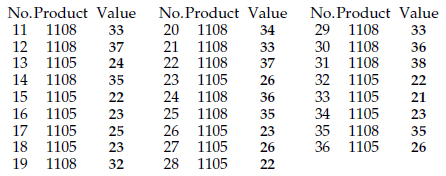Figure 1:  26 sequential measurements from Unit 11

Every so often, a sample is taken from the production stream and its final characteristic is measured. Figure 1 shows the sample number, the product type, and the measurement value for 26 consecutive samples from Unit 11. We shall use the 26 values of figure 1 to construct product specific charts for Products 1105 and 1108.

Product 1105 has an average value of 23.54 and an average moving range of 2.17. The target value for Product 1105 is 24.0. Product 1108 has an average value of 34.92 and an average moving range of 2.33. The target value for Product 1108 is 35.0 Both charts in figure 2 show predictable and reasonably on-target operation.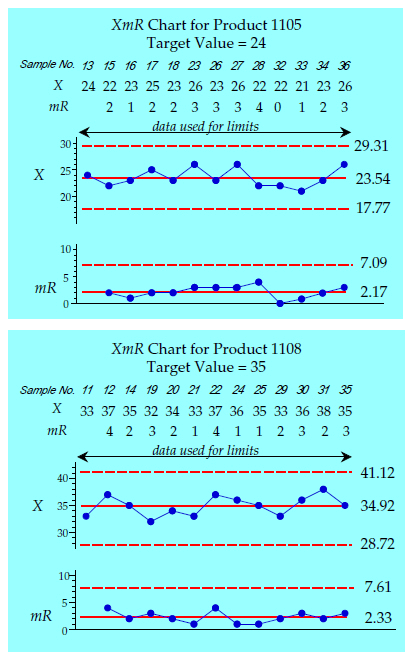Figure 2:  Product specific charts for Products 1105 and 1108

These product specific charts provide report cards that may be used for quality assurance with each product. Here we see that all of the Product 1105 samples appear to have come from one grand lot, and that all of the Product 1108 samples also appear to have come from another grand lot. However, since these charts fragment the running record of the production process they will be of little use to those who have to run the production process. Production personnel will need a different way of charting these data before they will be able to visualize the production process in real time.

How many data? When operating with short production runs it may take some time to accumulate data for some products. The product specific charts above have baselines of 13 values each. When possible, baselines of 10 to 20 values for each product specific chart are preferred. However, when necessary, limits may be computed using as few as five values per product.

The data above could be placed on a zed chart. However, when it is feasible, a difference chart is easier to use. To decide if a difference chart is feasible we need to know if the different products produced on Unit 11 have reasonably similar amounts of variation. And this is where we use an ANOMmR chart (pronounced a-nom-m-r).

### ANOMmR charts

The question of interest here is whether or not the different products display similar amounts of variation. But the ANOMmR chart is designed to identify detectable differences in variation from product to product. Therefore, we need to be comfortable that a finding of "no detectable difference" is equivalent to "the products display reasonably similar amounts of variation." To this end, we set the bar for detecting differences low by using a large alpha level.

If we have reason to believe the variation is resident in the product process itself, and that therefore all of the products should have the same variation, we might use a 5-percent alpha level with our ANOMmR chart.

If we have reason to believe that the variation might depend upon which product is being produced, or if we simply do not know what to expect, then we should use a 10-percent alpha level with the ANOMmR chart.

The baseline period for Product 1105 in figure 2 contains k = 13 values and has an average moving range of 2.17. The baseline period for Product 1108 contains k = 13 values and has an average moving range of 2.33. While these two values are so similar that we can be reasonably certain that the two products have a similar amount of variation, this is not always the case.

A rigorous comparison can be made between m average moving ranges using the analysis of mean moving ranges (ANOMmR). Let k denote the number of original data in the baseline period for each product. ANOMmR requires k to be the same for each product. Here k = 13.

The "grand average moving range" is the average of the average moving ranges from the m product specific charts. Here the grand average moving range value is 2.25. From the ANOMmR tables at the end of this article our 10-percent scaling factors for k = 13 and m = 2 are 0.700 and 1.300. The upper and lower ANOMmR limits are found by multiplying the grand average moving range of 2.25 by each of these scaling factors. The resulting limits for the ANOMmR chart in figure 3 have an overall risk of a false alarm of 10 percent.Figure 3: ANOMmR for Unit 11

With both average moving ranges falling inside the limits in figure 3 we can say that there is no detectable difference between the variation for Product 1105 and the variation for Product 1108. Having used a 10-percent alpha level we have made the analysis very sensitive. So the finding of no detectable difference is equivalent to saying the two products display reasonably similar amounts of variation and we can track the production process using a difference chart.

### Difference charts

Difference charts allow us to visualize the underlying production process even though it is used for short runs of different products. (Difference charts for individual values are also known as "X-nominal charts," and "X-target charts.") The "nominal value" which is subtracted from each observed value will be specific to each product. This nominal value may be either the historic average for that product, or a target value for that product.

When we force the central line for a difference chart to be zero we end up with a chart that simultaneously examines the process to see if it is operating predictably and on-target. With a zero central line the three-sigma limits for the difference chart are: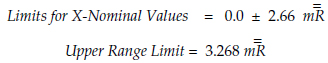Where the grand average moving range in these formulas is the central line from the ANOMmR chart (2.25 in this example). Figure 4 shows the data for Samples 37 to 60. The product specific charts including these data are shown in figures 5 and 6, while the difference chart for Unit 11, beginning with Sample 37, is shown in figure 7.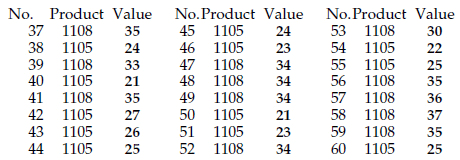Figure 4:  Samples 37 to 60 for Unit 11Figure 5: Product specific chart for Product 1105, Samples 13 to 60Figure 6: Product specific chart for Product 1108, Samples 11 to 59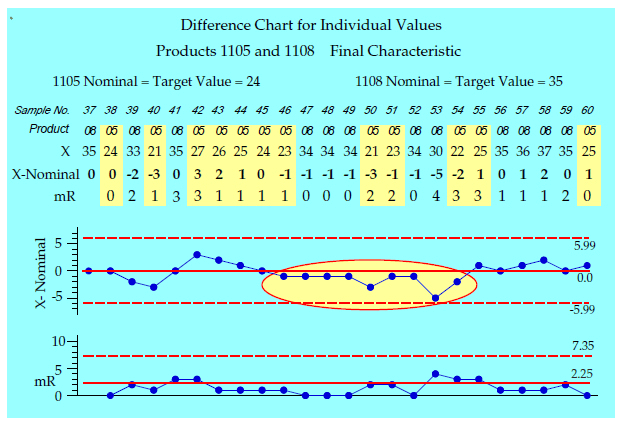Figure 7: Difference chart for Unit 11, Samples 37 to 60

The difference chart shows the underlying process in spite of the known changes in aim. Here we see a run below the central line for samples 46 to 54. This suggests that some assignable cause was affecting the values of both products during this period. This run does not show up in the charts in figures 5 and 6 for two reasons: First because these charts fragment the running record of the underlying process, and second because these two charts have central lines defined by the baseline averages rather than the nominal target values.

When investigating a suspicious point you should always begin by checking the computation of the difference. Subtracting off the wrong value is a quick way to create a false alarm. This is why it is a good idea to include the raw data on a difference chart.

The judgment that the multiple products produced on Unit 11 all displayed a similar amount of variation is what allows us to compute one set of limits for the difference chart. When the variation differs from product to product the difference chart will be inappropriate, and you will need to use a zed chart as described last month in "Short Run SPC, Part 1."

### ANOMmR for Unit 12

Last month the data from Unit 12 was used to illustrate the zed chart. Product 1201 had an average moving range of 5.07 units from a baseline of k = 15 original values (and 14 moving ranges). Product 1202 had an average moving range of 2.36 units from a baseline of k = 15 original values (and 14 moving ranges). Thus, the grand average moving range here is 3.71 units.

When comparing m = 2 average moving ranges from baselines with k = 15 individual values we find the 5-percent ANOMmR scaling factors of 0.671 and 1.329 from the table at the end of this article. Multiplying the grand average moving range of 3.71 by these scaling factors results in the chart in figure 8.Figure 8: ANOMmR for Unit 12

With the average moving ranges falling outside the limits in figure 8 we can say that there is a detectable difference between the variation for Product 1201 and the variation for Product 1202. Dividing by 1.128 we find Sigma(X) values of 4.49 and 2.09 respectively. This detectable difference means that we should not try to use a difference chart to track Unit 12. The zed chart created last month is the proper approach for these data.

Trying to use a difference chart for Unit 12 will shift the points around in an artificial manner. Those products with smaller amounts of variation will be shifted back toward the central line while those products with larger amounts of variation will be shifted away from the central line. This can be seen in figure 9 where the zed chart for Unit 12 is compared with a difference chart for the same values. (The nominal values for 1201 and 1202 are 19 and 8 respectively.)Figure 9: Zed chart and difference chart for Unit 12

While there is some parallelism between the two charts, they tell different stories when interpreted point by point. All of the Product 1201 points have been shifted away from the central line on the difference chart relative to their positions on the zed chart. And all of the Product 1202 points have been shifted toward the central line.

Thus, while Batches 76 and 81 both have zed scores of 2.4, the difference chart has Batch 76 above the upper limit while Batch 81 is only half-way in between the central line and the upper limit!

Likewise, Batches 91 and 92 have zed scores of -1.9 and -1.8, but on the difference chart Batch 92 is twice as far from the central line as Batch 91!

This shifting of different products by different amounts is an unavoidable consequence of using an effective Sigma(X) value of 3.29 on the difference chart, when the appropriate Sigma(X) values are 4.49 and 2.09 respectively. Thus, the result of using a difference chart when a zed chart is needed will be an increased number of both false alarms and missed signals.

### Summary

If the products being made on a common production line display reasonably similar amounts of variation, the production process may be visualized by a difference chart. However, if we use a difference chart when the variation differs markedly from product to product our difference chart will have an excess number of false alarms and missed signals.

Zed charts may always be used with streams of individual values representing different products produced on the same operating unit. They work whether or not the variation differs from product to product. If an ANOMmR chart with a 5- or 10-percent alpha level finds detectable differences in variation between the products then a zed chart is needed.

As will be illustrated in Part Three, there are correct and incorrect ways to create zed charts, so when using software be careful that the correct computations are used.

For a 1-percent ANOMmR table see "When Are Instruments Equivalent, Part 2," by Donald J. Wheeler and James Beagle III, Quality Digest, May 6, 2019.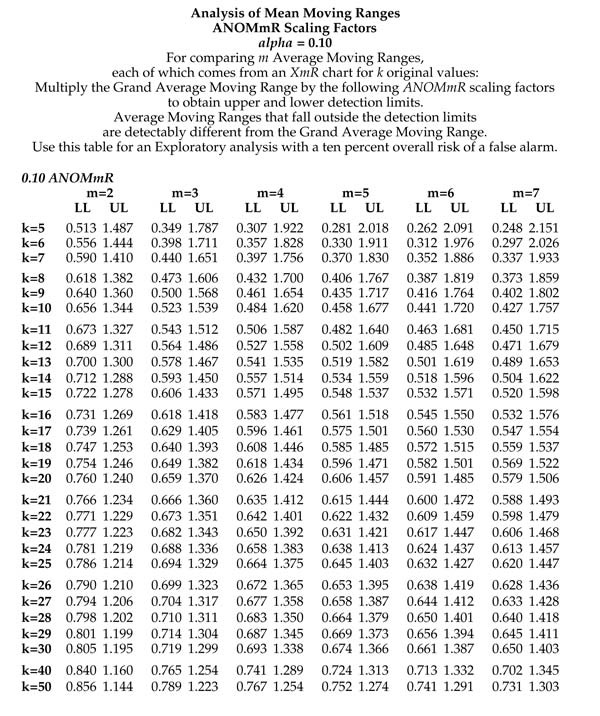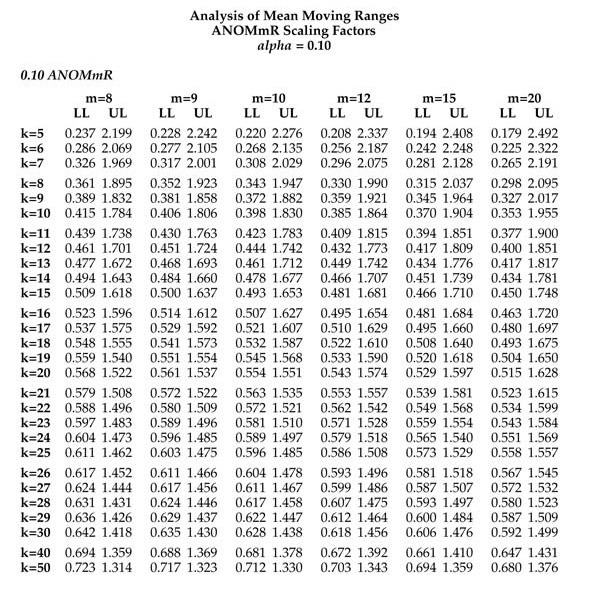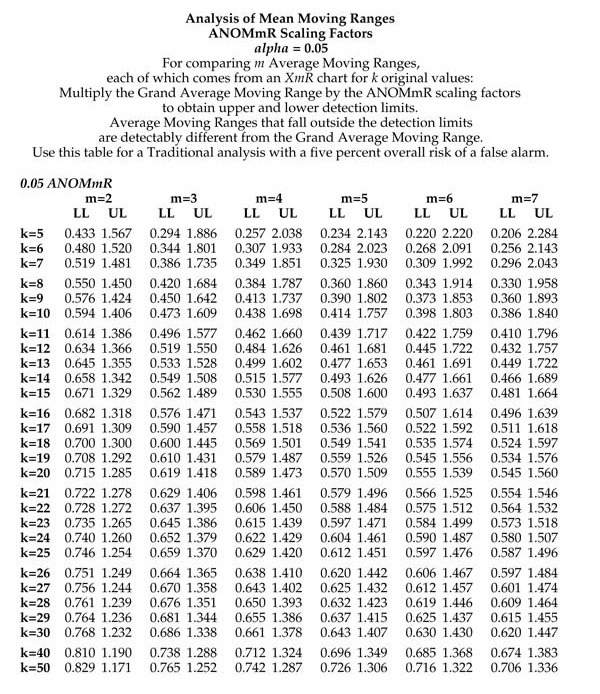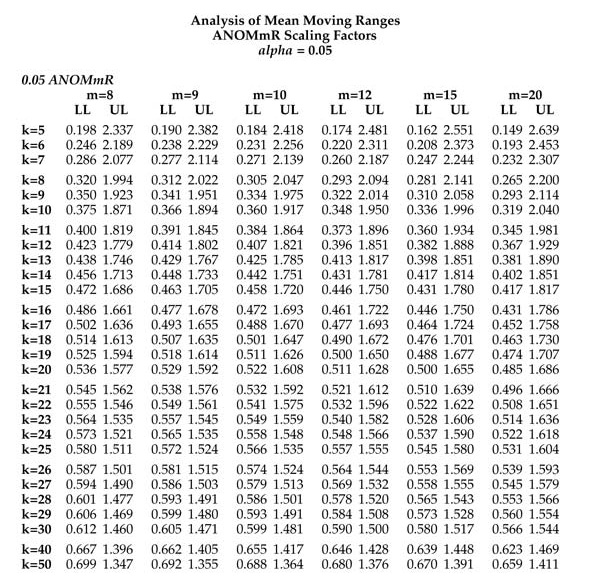### Discuss